Documentation

comm.LinearEqualizer

Equalize modulated signals using linear filtering

Description

The comm.LinearEqualizer System object™ uses a linear filter tap delay line with a weighted sum to equalize modulated signals transmitted through a dispersive channel. The equalizer object adaptively adjusts tap weights based on the selected algorithm. For more information, see Algorithms.

To equalize modulated signals using a linear filter:

1. Create the comm.LinearEqualizer object and set its properties.

2. Call the object with arguments, as if it were a function.

To learn more about how System objects work, see What Are System Objects? (MATLAB).

Creation

Description

example

lineq = comm.LinearEqualizer creates a linear equalizer System object to adaptively equalize a signal.

example

lineq = comm.LinearEqualizer(Name,Value) sets properties using one or more name-value pairs. For example, comm.LinearEqualizer('Algorithm','RLS') configures the equalizer object to update tap weights using the recursive least squares (RLS) algorithm. Enclose each property name in quotes.

Properties

expand all

Unless otherwise indicated, properties are nontunable, which means you cannot change their values after calling the object. Objects lock when you call them, and the release function unlocks them.

If a property is tunable, you can change its value at any time.

For more information on changing property values, see System Design in MATLAB Using System Objects (MATLAB).

Adaptive algorithm used for equalization, specified as one of these values:

Data Types: char | string

Number of equalizer taps, specified as a positive integer.

Data Types: double

Step size used by the adaptive algorithm, specified as a positive scalar. Increasing the step size reduces the equalizer convergence time but causes the equalizer output estimates to be less stable.

Tip

To determine the maximum step size allowed, use the maxstep object function.

Tunable: Yes

Dependencies

To enable this property, set Algorithm to 'LMS' or 'CMA'.

Data Types: double

Forgetting factor used by the adaptive algorithm, specified as a scalar in the range (0, 1]. Decreasing the forgetting factor reduces the equalizer convergence time but causes the equalizer output estimates to be less stable.

Tunable: Yes

Dependencies

To enable this property, set Algorithm to 'RLS'.

Data Types: double

Initial inverse correlation matrix, specified as a scalar or an NTaps-by-NTaps matrix. NTaps is equal to the NumTaps property value. If you specify InitialInverseCorrelationMatrix as a scalar, a, the equalizer sets the initial inverse correlation matrix to a times the identity matrix: a(eye(NTaps)).

Tunable: Yes

Dependencies

To enable this property, set Algorithm to 'RLS'.

Data Types: double

Signal constellation, specified as a vector. The default value is a QPSK constellation generated using this code: pskmod(0:3,4,pi/4).

Tunable: Yes

Data Types: double

Reference tap, specified as a positive integer less than or equal to the NumTaps property value. The equalizer uses the reference tap location to track the main energy of the channel.

Data Types: double

Input signal delay in samples relative to the reset time of the equalizer, specified as a nonnegative integer. If the input signal is a vector of length greater than 1, then the input delay is relative to the start of the input vector. If the input signal is a scalar, then the input delay is relative to the first call of the System object and to the first call of the System object after calling the release or reset object function.

Data Types: double

Number of input samples per symbol, specified as a positive integer. Setting this property to any number greater than one effectively creates a fractionally spaced equalizer. For more information, see Symbol Tap Spacing.

Data Types: double

Enable training control input, specified as false or true. Setting this property to true enables the equalizer training flag input tf.

Tunable: Yes

Data Types: logical

Update tap weights when not training, specified as true or false. If this property is set to true, the System object uses decision directed mode to update equalizer tap weights. If this property is set to false, the System object keeps the equalizer tap weights unchanged after training.

Tunable: Yes

Data Types: logical

Source of adapt tap weights request, specified as one of these values:

• 'Property' — Specify this value to use the AdaptWeights property to control when the System object adapts tap weights.

• 'Input port' — Specify this value to use the aw input to control when the System object adapts tap weights.

Dependencies

To enable this property, set Algorithm to 'CMA'.

Data Types: char | string

Adapt tap weights, specified as true or false. If this property is set to true, the System object updates the equalizer tap weights. If this property is set to false, the System object keeps the equalizer tap weights unchanged.

Tunable: Yes

Dependencies

To enable this property, set AdaptWeightsSource to 'Property' and set AdaptAfterTraining to true.

Data Types: logical

Source for initial tap weights, specified as

• 'Auto' — Initialize the tap weights to the algorithm-specific default values, as described in the InitialWeights property.

• 'Property' — Initialize the tap weights using the InitialWeights property value.

Data Types: char | string

Initial tap weights used by the adaptive algorithm, specified as a scalar or vector. The default is 0 when the Algorithm property is set to 'LMS' or 'RLS'. The default is [0;0;1;0;0] when the Algorithm property is set to 'CMA'.

If you specify InitialWeights as a vector, the vector length must be equal to the NumTaps property value. If you specify InitialWeights as a scalar, the equalizer uses scalar expansion to create a vector of length NumTaps with all values set to InitialWeights.

Tunable: Yes

Dependencies

To enable this property, set InitialWeightsSource to 'Property'.

Data Types: double

Tap weight update period in symbols, specified as a positive integer. The equalizer updates the tap weights after processing this number of symbols.

Data Types: double

Usage

Description

example

y = lineq(x,tsym) equalizes input signal x by using training symbols tsym. The output is the equalized symbols. To enable this syntax, set the Algorithm property to 'LMS' or 'RLS'.

example

y = lineq(x,tsym,tf) also specifies training flag tf. The System object starts training when tf changes from false to true (at the rising edge). The System object trains until all symbols in tsym are processed. The input tsym is ignored when tf is false. To enable this syntax, set the Algorithm property to 'LMS' or 'RLS' and TrainingFlagInputPort property to true.

example

y = lineq(x) equalizes input signal x. To enable this syntax, set the Algorithm property to 'CMA'.

example

y = lineq(x,aw) also specifies adapts weights flag aw. The System object adapts the equalizer tap weights when aw is true. If aw is false, the System object keeps the weights unchanged. To enable this syntax, set the Algorithm property to 'CMA' and AdaptWeightsSource property to 'Input port'.

example

[y,err] = lineq(___) also returns error signal err using input arguments from any of the previous syntaxes.

example

[y,err,weights] = lineq(___) also returns weights, the tap weights from the last tap weight update, using input arguments from any of the previous syntaxes.

Input Arguments

expand all

Input signal, specified as a column vector. The input signal vector length must be equal to an integer multiple of the InputSamplesPerSymbol property value. For more information, see Symbol Tap Spacing.

Data Types: double
Complex Number Support: Yes

Training symbols, specified as a column vector of length less than or equal to the length of input x. The input tsym is ignored when tf is false.

Dependencies

To enable this argument, set the Algorithm property to 'LMS' or 'RLS'.

Data Types: double
Complex Number Support: Yes

Training flag, specified as true or false. The System object starts training when tf changes from false to true (at the rising edge). The System object trains until all symbols in tsym are processed. The input tsym is ignored when tf is false.

Dependencies

To enable this argument, set the Algorithm property to 'LMS' or 'RLS' and TrainingFlagInputPort property to true.

Data Types: logical

Adapt weights flag, specified as true or false. If aw is true, the System object adapts weights. If aw is false, the System object keeps the weights unchanged.

Dependencies

To enable this argument, set the Algorithm property to 'CMA' and AdaptWeightsSource property to 'Input port'.

Data Types: logical

Output Arguments

expand all

Equalized symbols, returned as a column vector that has the same length as input signal x.

Error signal, returned as a column vector that has the same length as input signal x.

Tap weights, returned as a column vector that has NumTaps elements. weights contains the tap weights from the last tap weight update.

Object Functions

To use an object function, specify the System object as the first input argument. For example, to release system resources of a System object named obj, use this syntax:

release(obj)

expand all

 isLocked Determine if System object is in use clone Create duplicate System object info Characteristic information about the equalizer object maxstep Maximum step size for LMS equalizer convergence mmseweights Linear equalizer MMSE tap weights
 step Run System object algorithm release Release resources and allow changes to System object property values and input characteristics reset Reset internal states of System object

Examples

expand all

Create a BPSK modulator and an equalizer System object™, specifying a linear LMS equalizer having eight taps and a step size of 0.03.

bpsk = comm.BPSKModulator;
eqlms = comm.LinearEqualizer('Algorithm','LMS','NumTaps',8,'StepSize',0.03);

Change the reference tap index of the equalizer.

eqlms.ReferenceTap = 4;

Build a set of test data. Receive the data by convolving the signal.

x = bpsk(randi([0 1],1000,1));
rxsig = conv(x,[1 0.8 0.3]);

Use maxstep to find the maximum permitted step size.

mxStep = maxstep(eqlms,rxsig)
mxStep = 0.1384

Equalize the received signal. Use the first 200 symbols as the training sequence.

y = eqlms(rxsig,x(1:200));

Apply linear equalization using the least mean squares (LMS) algorithm to recover QPSK symbols passed through a multipath AWGN channel.

Initialize simulation variables.

M = 4; % QPSK
numSymbols = 10000;
numTrainingSymbols = 1000;
chtaps = [1 0.5*exp(1i*pi/6) 0.1*exp(-1i*pi/8)];

Generate QPSK-modulated symbols. Apply multipath channel filtering and AWGN impairments to the symbols.

data = randi([0 M-1],numSymbols,1);
tx = pskmod(data,M,pi/4);
rx = awgn(filter(chtaps,1,tx),25,'measured');

Create a linear equalizer System object and display the default configuration. Adjust the reference tap to 1. Check the maximum permitted step size. Equalize the impaired symbols.

eq = comm.LinearEqualizer
eq =
comm.LinearEqualizer with properties:

Algorithm: 'LMS'
NumTaps: 5
StepSize: 0.0100
Constellation: [1x4 double]
ReferenceTap: 3
InputDelay: 0
InputSamplesPerSymbol: 1
TrainingFlagInputPort: false
InitialWeightsSource: 'Auto'
WeightUpdatePeriod: 1

eq.ReferenceTap = 1;

mxStep = maxstep(eq,rx)
mxStep = 0.3154
[y,err,weights] = eq(rx,tx(1:numTrainingSymbols));

Plot the constellation of the impaired and equalized symbols.

constell = comm.ConstellationDiagram('NumInputPorts',2);
constell(rx,y)Plot the equalizer error signal and compute the error vector magnitude (EVM) of the equalized symbols.

plot(abs(err))
grid on; xlabel('Symbols'); ylabel('|e|');title('Equalizer Error Signal')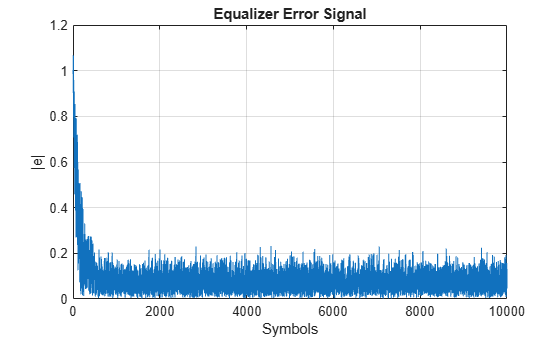errevm = comm.EVM;
evm = errevm(tx,y)
evm = 11.7710

Plot the equalizer tap weights.

subplot(3,1,1);
stem(real(weights)); ylabel('real(weights)'); xlabel('Tap'); grid on; axis([0 6 -0.5 1])
title('Equalizer Tap Weights')
subplot(3,1,2);
stem(imag(weights)); ylabel('imag(weights)'); xlabel('Tap'); grid on; axis([0 6 -0.5 1])
subplot(3,1,3);
stem(abs(weights)); ylabel('abs(weights)'); xlabel('Tap'); grid on; axis([0 6 -0.5 1])Demonstrate linear equalization by using the least mean squares (LMS) algorithm to recover QPSK symbols passed through an AWGN channel. Apply different equalizer training schemes and show the symbol error magnitude.

System Setup

Simulate a QPSK-modulated system subject to AWGN. Transmit packets composed of 200 training symbols and 1800 random data symbols. Configure a linear LMS equalizer to recover the packet data.

M = 4;
numTrainSymbols = 200;
numDataSymbols = 1800;
SNR = 20;
trainingSymbols = pskmod(randi([0 M-1],numTrainSymbols,1),M,pi/4);
numPkts = 10;
lineq = comm.LinearEqualizer('Algorithm','LMS', ...
'NumTaps',5,'ReferenceTap',3,'StepSize',0.01);

Train the Equalizer at the Beginning of Each Packet With Reset

Use prepended training symbols when processing each packet. After processing each packet, reset the equalizer. This reset forces the equalizer to train the taps with no previous knowledge. Equalizer error signal plots for the first, second, and last packet show higher symbol errors at the start of each packet.

jj = 1;
figure
for ii = 1:numPkts
b = randi([0 M-1],numDataSymbols,1);
dataSym = pskmod(b,M,pi/4);
packet = [trainingSymbols;dataSym];
rx = awgn(packet,SNR);
[~,err] = lineq(rx,trainingSymbols);
reset(lineq)
if (ii ==1 || ii == 2 ||ii == numPkts)
subplot(3,1,jj)
plot(abs(err))
title(['Packet # ',num2str(ii)])
xlabel('Symbols')
ylabel('Error Magnitude')
axis([0,length(packet),0,1])
grid on;
jj = jj+1;
end
endTrain the Equalizer at the Beginning of Each Packet Without Reset

Process each packet using prepended training symbols. Do not reset the equalizer after each packet is processed. By not resetting after each packet, the equalizer retains tap weights from training prior packets. Equalizer error signal plots for the first, second, and last packet show that after the initial training on the first packet, subsequent packets have less symbol errors at the start of each packet.

release(lineq)
jj = 1;
figure
for ii = 1:numPkts
b = randi([0 M-1],numDataSymbols,1);
dataSym = pskmod(b,M,pi/4);
packet = [trainingSymbols;dataSym];
channel = 1;
rx = awgn(packet*channel,SNR);
[~,err] = lineq(rx,trainingSymbols);
if (ii ==1 || ii == 2 ||ii == numPkts)
subplot(3,1,jj)
plot(abs(err))
title(['Packet # ',num2str(ii)])
xlabel('Symbols')
ylabel('Error Magnitude')
axis([0,length(packet),0,1])
grid on;
jj = jj+1;
end
endTrain the Equalizer Periodically

Systems with signals subject to time-varying channels require periodic equalizer training to maintain lock on the channel variations. Specify a system that has 200 symbols of training for every 1800 data symbols. Between training, the equalizer does not update tap weights. The equalizer processes 200 symbols per packet.

Rs = 1e6;
fd = 20;
spp = 200; % Symbols per packet
b = randi([0 M-1],numDataSymbols,1);
dataSym = pskmod(b,M,pi/4);
packet = [trainingSymbols; dataSym];
stream = repmat(packet,10,1);
tx = (0:length(stream)-1)'/Rs;
channel = exp(1i*2*pi*fd*tx);
rx = awgn(stream.*channel,SNR);

Set the AdaptAfterTraining property to false to stop the equalizer tap weight updates after the training phase.

release(lineq)
lineq =
comm.LinearEqualizer with properties:

Algorithm: 'LMS'
NumTaps: 5
StepSize: 0.0100
Constellation: [1x4 double]
ReferenceTap: 3
InputDelay: 0
InputSamplesPerSymbol: 1
TrainingFlagInputPort: false
InitialWeightsSource: 'Auto'
WeightUpdatePeriod: 1

Equalize the impaired data. Plot the angular error from the channel, the equalizer error signal, and signal constellation. As the channel varies, the equalizer output does not remove the channel effects. The output constellation rotates out of sync, resulting in bit errors.

[y,err] = lineq(rx,trainingSymbols);

figure
subplot(2,1,1)
plot(tx, unwrap(angle(channel)))
xlabel('Time (sec)')
title('Angular Error Over Time')
subplot(2,1,2)
plot(abs(err))
xlabel('Symbols')
ylabel('Error Magnitude')
grid on
title('Time-Varying Channel Without Retraining')scatterplot(y)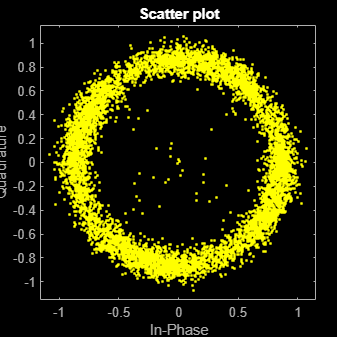Set the TrainingInputPort property to true to configure the equalizer to retrain the taps when signaled by the trainFlag input. The equalizer trains only when trainFlag is true. After every 2000 symbols, the equalizer retrains the taps and keeps lock on variations of the channel. Plot the angular error from the channel, equalizer error signal, and signal constellation. As the channel varies, the equalizer output removes the channel effects. The output constellation does not rotate out of sync and bit errors are reduced.

release(lineq)
lineq.TrainingFlagInputPort = true;
symbolCnt = 0;
numPackets = length(rx)/spp;
trainFlag = true;
trainingPeriod = 2000;
eVec = zeros(size(rx));
yVec = zeros(size(rx));
for p=1:numPackets
[yVec((p-1)*spp+1:p*spp,1),eVec((p-1)*spp+1:p*spp,1)] = ...
lineq(rx((p-1)*spp+1:p*spp,1),trainingSymbols,trainFlag);
symbolCnt = symbolCnt + spp;
if symbolCnt >= trainingPeriod
trainFlag = true;
symbolCnt = 0;
else
trainFlag = false;
end
end
figure
subplot(2,1,1)
plot(tx, unwrap(angle(channel)))
xlabel('t (sec)')
title('Angular Error Over Time')
subplot(2,1,2)
plot(abs(eVec))
xlabel('Symbols')
ylabel('Error Magnitude')
grid on
title('Time-Varying Channel With Retraining')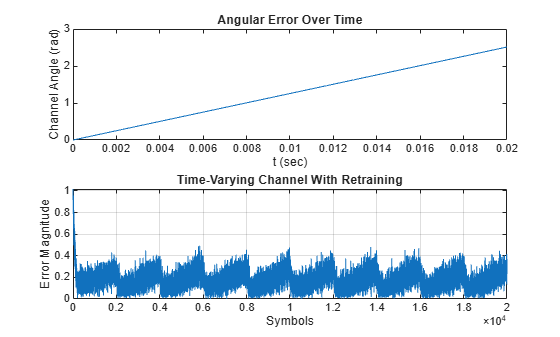scatterplot(yVec)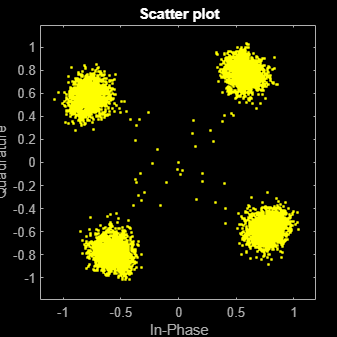Simulate a system with delay between the transmitted symbols and received samples. Typical systems have transmitter and receiver filters that result in a delay. This delay must be accounted for to synchronize the system. In this example, the system delay is introduced without transmit and receive filters. Linear equalization, using the least mean squares (LMS) algorithm, recovers QPSK symbols.

Initialize simulation variables.

M = 4; % QPSK
numSymbols = 10000;
numTrainingSymbols = 1000;
mpChan = [1 0.5*exp(1i*pi/6) 0.1*exp(-1i*pi/8)];
systemDelay = dsp.Delay(20);
snr = 24;

Generate QPSK-modulated symbols. Apply multipath channel filtering, a system delay, and AWGN to the transmitted symbols.

data = randi([0 M-1],numSymbols,1);
tx = pskmod(data,M,pi/4); % OQPSK
delayedSym = systemDelay(filter(mpChan,1,tx));
rx = awgn(delayedSym,snr,'measured');

Create equalizer and EVM System objects. The equalizer System object specifies a linear equalizer that uses the LMS algorithm.

lineq = comm.LinearEqualizer('Algorithm','LMS', ...
'NumTaps',9,'ReferenceTap',5);
evm = comm.EVM('ReferenceSignalSource', ...
'Estimated from reference constellation');

Equalize Without Adjusting Input Delay

Equalize the received symbols.

[y1,err1,wts1] = lineq(rx,tx(1:numTrainingSymbols,1));

Find the delay between the received symbols and the transmitted symbols by using the finddelay function.

rxDelay = finddelay(tx,rx)
rxDelay = 20

Display the equalizer information. The latency value indicates the delay introduced by the equalizer. Calculate the total delay as the sum of rxDelay and the equalizer latency.

eqInfo = info(lineq)
eqInfo = struct with fields:
Latency: 4

totalDelay = rxDelay + eqInfo.Latency;

Until the equalizer output converges, the symbol error rate is high. Plot the error output, err1, to determine when the equalized output converges.

plot(abs(err1))
xlabel('Symbols')
ylabel('Error Magnitude')
title('Equalizer Error Signal')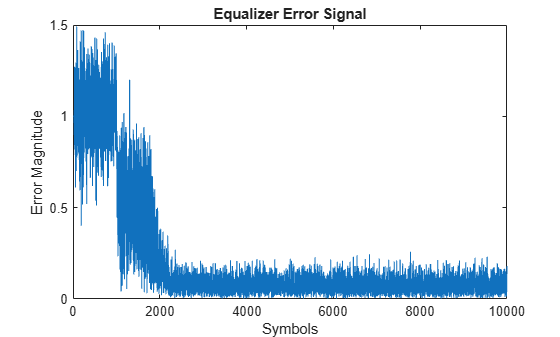The plot shows excessive errors beyond the 1000 symbols training period. When demodulating symbols and computing symbol errors, to account for the unconverged output and the system delay between the equalizer output and transmitted symbols, skip the first 2000 symbols.

dataRec1 = pskdemod(y1(2000+totalDelay:end),M,pi/4);
symErrWithDelay = symerr(data(2000:end-totalDelay),dataRec1)
symErrWithDelay = 5999
evmWithDelay = evm(y1)
evmWithDelay = 29.5795

The error rate and EVM are high because the receive delay was not accounted for in the equalizer System object.

Adjust Input Delay in Equalizer

Equalize the received data by using the delay value to set the InputDelay property. Because InputDelay is a nontunable property, you must release the lineq System object to reconfigure the InputDelay property. Equalize the received symbols.

release(lineq)
lineq.InputDelay = rxDelay
lineq =
comm.LinearEqualizer with properties:

Algorithm: 'LMS'
NumTaps: 9
StepSize: 0.0100
Constellation: [1x4 double]
ReferenceTap: 5
InputDelay: 20
InputSamplesPerSymbol: 1
TrainingFlagInputPort: false
InitialWeightsSource: 'Auto'
WeightUpdatePeriod: 1

[y2,err2,wts2] = lineq(rx,tx(1:numTrainingSymbols,1));

Plot the tap weights and equalized error magnitude. A stem plot shows the equalizer tap weights before and after the system delay is removed. A 2-D line plot shows the slower equalizer convergence for the delayed signal as compared to the signal with the delay removed.

subplot(2,1,1)
stem([real(wts1),real(wts2)])
xlabel('Taps')
ylabel('Tap Weight Real')
legend('rxDelayed','rxDelayRemoved')
grid on
subplot(2,1,2)
stem([imag(wts1),imag(wts2)])
xlabel('Taps')
ylabel('Tap Weight Imaginary')
legend('rxDelayed','rxDelayRemoved')
grid on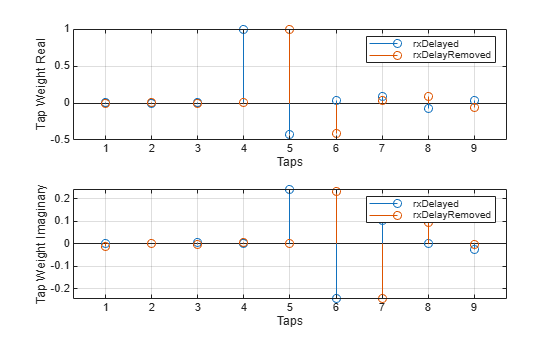figure
plot([abs(err1),abs(err2)])
xlabel('Symbols')
ylabel('Error Magnitude')
legend('rxDelayed','rxDelayRemoved')
grid on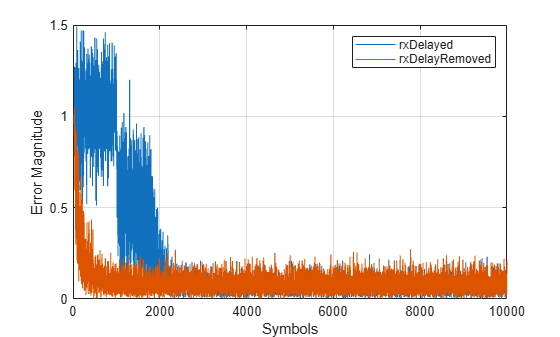Plot error output of the equalized signals, rxDelayed and rxDelayRemoved. For the signal that has the delay removed, the equalizer converges during the 1000 symbol training period. When demodulating symbols and computing symbol errors, to account for the unconverged output and the system delay between the equalizer output and transmitted symbols, skip the first 500 symbols. Reconfiguring the equalizer to account for the system delay enables better equalization of the signal, and reduces symbol errors and the EVM.

eqInfo = info(lineq)
eqInfo = struct with fields:
Latency: 4

totalDelay = rxDelay + eqInfo.Latency;
dataRec2 = pskdemod(y2(500+totalDelay:end),M,pi/4);
symErrDelayRemoved = symerr(data(500:end-totalDelay),dataRec2)
symErrDelayRemoved = 0
evmDelayRemoved = evm(y2(500+totalDelay:end))
evmDelayRemoved = 9.4435

Recover QPSK symbols with a linear equalizer by using the constant modulus algorithm (CMA) and EVM-based taps training. When using blind equalizer algorithms, such as CMA, train the equalizer taps by using the AdaptWeights property to start and stop training. Helper functions are used to generate plots and apply phase correction.

Initialize system variables.

rng(123456);
M = 4; % QPSK
numSymbols = 100;
numPackets = 5000;
raylChan = comm.RayleighChannel('PathDelays',[0 1], ...
'AveragePathGains',[0 -12],'MaximumDopplerShift',1e-5);
SNR = 50;

Create the equalizer and EVM System objects. The equalizer System object specifies a linear equalizer by using the CMA adaptive algorithm. Call the helper function to initialize figure plots.

lineq = comm.LinearEqualizer('Algorithm','CMA', ...
'NumTaps',5,'ReferenceTap',3, ...
lineq =
comm.LinearEqualizer with properties:

Algorithm: 'CMA'
NumTaps: 5
StepSize: 0.0300
Constellation: [1x4 double]
ReferenceTap: 3
InputSamplesPerSymbol: 1
InitialWeightsSource: 'Auto'
WeightUpdatePeriod: 1

info(lineq)
ans = struct with fields:
Latency: 2

evm = comm.EVM('ReferenceSignalSource', ...
'Estimated from reference constellation');

Equalization Loop

To implement the equalization loop:

1. Generate PSK data packets.

2. Apply Rayleigh fading and AWGN to the transmission data.

3. Apply equalization to the received data and phase correction to the equalizer output.

4. Estimate the EVM and toggle the adaptWeights flag to true or false based on the EVM level.

5. Update the figure plots.

for p=1:numPackets
data = randi([0 M-1],numSymbols,1);
tx = pskmod(data,M,pi/4);
rx = awgn(raylChan(tx),SNR);
rxDelay = finddelay(rx,tx);
y = phaseCorrection(y);
evmEst = evm(y);
adaptWeights = (evmEst > 20);

end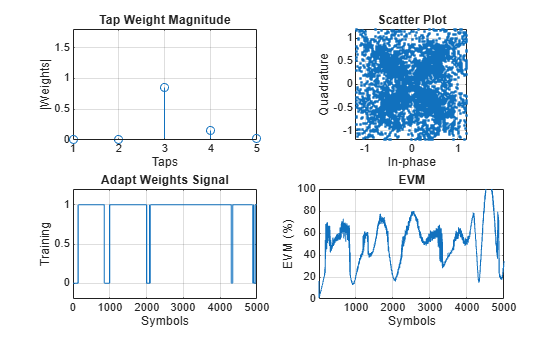rxDelay
rxDelay = 0

The figure plots show that, as the EVM varies, the equalizer toggles in and out of decision-directed weight adaptation mode.

Helper Functions

This helper function initializes figures that show a quad plot of simulation results.

function [errPlot,evmPlot,scatter,adaptState] = initFigures(numPkts,lineq)
yVec = nan(numPkts,1);
evmVec = nan(numPkts,1);
wVec = zeros(lineq.NumTaps,1);

figure
subplot(2,2,1)
evmPlot = stem(wVec);
grid on; axis([1 lineq.NumTaps 0 1.8])
xlabel('Taps'); ylabel('|Weights|'); title('Tap Weight Magnitude')

subplot(2,2,2)
scatter = plot(yVec, '.');
axis square; axis([-1.2 1.2 -1.2 1.2]); grid on
xlabel('In-phase'); ylabel('Quadrature'); title('Scatter Plot');
subplot(2,2,3)
grid on; axis([0 numPkts -0.2 1.2])
ylabel('Training'); xlabel('Symbols'); title('Adapt Weights Signal')
subplot(2,2,4)
errPlot = plot(evmVec);
grid on; axis([1 numPkts 0 100])
xlabel('Symbols'); ylabel('EVM (%)'); title('EVM')
end

This helper function updates figures.

function updateFigures(errPlot,evmPlot,scatSym, ...
persistent yVec evmVec adaptVec

if p == 1
yVec = nan(numFrames,1);
evmVec = nan(numFrames,1);
end

yVec(p) = y;
evmVec(p) = evmEst;

errPlot.YData = abs(evmVec);
evmPlot.YData = abs(w);
scatSym.XData = real(yVec);
scatSym.YData = imag(yVec);
drawnow limitrate
end

This helper function applies phase correction.

function y = phaseCorrection(y)
a = angle(y((real(y) > 0) & (imag(y) > 0)));
a(a < 0.1) = a(a < 0.1) + pi/2;
theta = mean(a) - pi/4;
y = y * exp(-1i*theta);
end

Recover QPSK symbols in fading environments with a linear equalizer, using the least mean squares (LMS) algorithm. Use the reset object function to equalize independent packets. Use helper functions to generate plots. This example also shows symbol-based processing and frame-based processing.

Setup

Initialize system variables, create an equalizer System object, and initialize the plot figures.

M = 4; % QPSK
numSym = 1000;
numTrainingSym = 100;
numPackets = 5;
numTaps = 9;
ttlNumSym = numSym + numTrainingSym;
raylChan = comm.RayleighChannel('PathDelays',[0 1], ...
'AveragePathGains',[0 -9], ...
'MaximumDopplerShift',0, ...
'PathGainsOutputPort',true);
SNR = 35;
rxVec = zeros(ttlNumSym,numPackets);
txVec = zeros(ttlNumSym,numPackets);
yVec = zeros(ttlNumSym,1);
eVec = zeros(ttlNumSym,1);

lineq1 = comm.LinearEqualizer('Algorithm','LMS', ...
'NumTaps',numTaps,'ReferenceTap',5, ...
'StepSize',0.01,'TrainingFlagInputPort',true);

[errPlot,wStem,hStem,scatPlot] = initFigures(ttlNumSym,lineq1, ...
raylChan.AveragePathGains);

Symbol-Based Processing

For symbol-based processing, provide one symbol at the input of the equalizer. Reset the equalizer state and channel after processing each packet.

for p = 1:numPackets
trainingFlag = true;
for q=1:ttlNumSym
data = randi([0 M-1],1,1);
tx = pskmod(data,M,pi/4);
[xc,pg] = raylChan(tx);
rx = awgn(xc,25);
[y,err,wts] = lineq1(rx,tx,trainingFlag);

Disable training after processing numTrainingSym training symbols.

if q == numTrainingSym
trainingFlag = false;
end
updateFigures(errPlot,wStem,hStem,scatPlot,err,wts,y,pg,q,ttlNumSym);
txVec(q,p) = tx;
rxVec(q,p) = rx;
end

After processing each packet, reset the channel System object to get a new realization of channel taps and the equalizer System object to restore the default taps weights.

reset(raylChan)
reset(lineq1)
endPacket-Based Processing

For packet-based processing, provide one packet at the input of the equalizer. Each packet contains ttlNumSym symbols. Because the training duration is less than the packet length, you do not need to specify the start-training input.

yVecPkt = zeros(ttlNumSym,numPackets);
errVecPkt = zeros(ttlNumSym,numPackets);
wgtVecPkt = zeros(numTaps,numPackets);
lineq2 = comm.LinearEqualizer('Algorithm','LMS', ...
'NumTaps',9,'ReferenceTap',6,'StepSize',0.01);
for p = 1:numPackets
[yVecPkt(:,p),errVecPkt(:,p),wgtVecPkt(:,p)] = ...
lineq2(rxVec(:,p),txVec(1:numTrainingSym,p));
for q=1:ttlNumSym
updateFigures(errPlot,wStem,hStem,scatPlot, ...
errVecPkt(q,p),wgtVecPkt(:,p),yVecPkt(q,p),pg,q,ttlNumSym);
end

After processing each packet, reset the channel System object to get a new realization of channel taps and the equalizer System object to restore the default taps weights.

reset(raylChan)
reset(lineq2)
endHelper Functions

The helper function initializes the figures.

function [errPlot,wStem,hStem,scatPlot] = initFigures(ttlNumSym,lineq,pg)
yVec = nan(ttlNumSym,1);
eVec = nan(ttlNumSym,1);
wVec = zeros(lineq.NumTaps,1);
figure;
subplot(2,2,1);
wStem = stem(wVec);
axis([1 lineq.NumTaps 0 1.8]); grid on
xlabel('Taps'); ylabel('|Weights|'); title('Tap Weight Magnitude')
subplot(2,2,2);
hStem = stem([0 abs(pg) 0]);
grid on;
xlabel('Taps'); ylabel('|Path Gain|'); title('Channel Path Gain Magnitude')
subplot(2,2,3);
errPlot = plot(eVec);
axis([1 ttlNumSym 0 1.2]); grid on
xlabel('Symbols'); ylabel('|Error Magnitude|'); title('Error Magnitude')
subplot(2,2,4);
scatPlot = plot(yVec,'.');
axis square; axis([-1.2 1.2 -1.2 1.2]); grid on;
xlabel('In-phase'); ylabel('Quadrature'); title(sprintf('Scatter Plot'));
end

This helper function updates the figures.

function updateFigures(errPlot,wStem,hStem,scatPlot, ...
err,wts,y,pg,p,ttlNumSym)
persistent yVec eVec
if p == 1
yVec = nan(ttlNumSym,1);
eVec = nan(ttlNumSym,1);
end
yVec(p) = y;
eVec(p) = abs(err);
errPlot.YData = abs(eVec);
wStem.YData = abs(wts);
hStem.YData = [0 abs(pg) 0];
scatPlot.XData = real(yVec);
scatPlot.YData = imag(yVec);
drawnow limitrate
end

Use the linear equalizer in nonadaptive mode. Use the mmseweights object function to calulate the minimum mean squared error (MMSE) solution and use the weights for the linear equalizer taps weights.

Initialize simulation variables.

M = 4; % QPSK
numSymbols = 10000;
numTrainingSymbols = 1000;
chtaps = [1 0.5*exp(1i*pi/6) 0.1*exp(-1i*pi/8)];
EbN0 = 20;

Generate QPSK modulated symbols. Apply delayed multipath channel filtering and AWGN impairments to the symbols.

data = randi([0 M-1], numSymbols, 1);
tx = pskmod(data, M, pi/4);
rx = awgn(filter(chtaps,1,tx),25,'measured');

Create a linear equalizer System object configured to use CMA algorithm, set the AdaptWeights property to false, and the InitialWeightsSource property to Property. Calculate the MMSE weights. Set the initial tap weights to the calculated MMSE weights. Equalize the impaired symbols.

eq =
comm.LinearEqualizer with properties:

Algorithm: 'CMA'
NumTaps: 5
StepSize: 0.0100
Constellation: [1x4 double]
InputSamplesPerSymbol: 1
InitialWeightsSource: 'Property'
InitialWeights: [5x1 double]
WeightUpdatePeriod: 1

wgts = mmseweights(eq,chtaps,EbN0)
wgts = 5×1 complex

0.0005 - 0.0068i
0.0103 + 0.0117i
0.9694 - 0.0019i
-0.3987 + 0.2186i
0.0389 - 0.1756i

eq.InitialWeights = wgts;

[y,err,weights] = eq(rx);

Plot constellation of impaired and equalized symbols.

constell = comm.ConstellationDiagram('NumInputPorts',2);
constell(rx,y)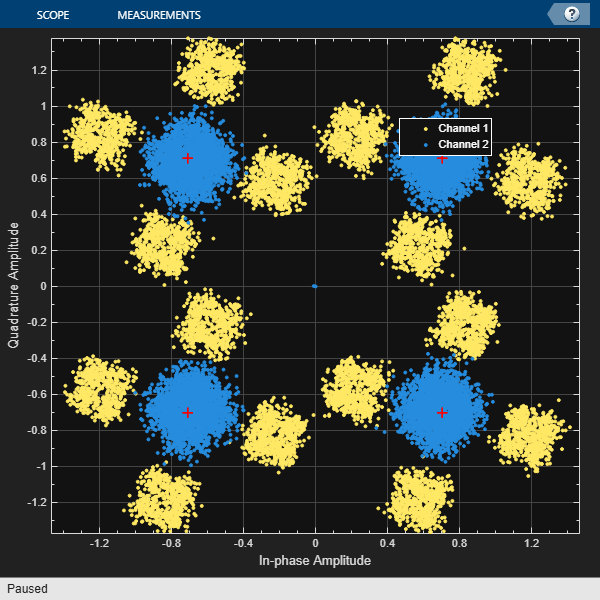Plot the equalizer error signal and compute the error vector magnitude of the equalized symbols.

plot(abs(err))
grid on; xlabel('Symbols'); ylabel('|e|')errevm = comm.EVM;
evm = errevm(tx,y)
evm = 140.6177

Plot equalizer tap weights.

subplot(3,1,1); stem(real(weights)); ylabel('real(weights)'); xlabel('Tap'); grid on; axis([1 8 -0.5 1])
line([eq.NumTaps+0.5 eq.NumTaps+0.5], [-0.5 1], 'Color', 'r', 'LineWidth', 1)
title('Equalizer Tap Weights')
subplot(3,1,2); stem(imag(weights)); ylabel('imag(weights)'); xlabel('Tap'); grid on; axis([1 8 -0.5 1])
line([eq.NumTaps+0.5 eq.NumTaps+0.5], [-0.5 1], 'Color', 'r', 'LineWidth', 1)
subplot(3,1,3); stem(abs(weights)); ylabel('abs(weights)'); xlabel('Tap'); grid on; axis([1 8 -0.5 1])
line([eq.NumTaps+0.5 eq.NumTaps+0.5], [-0.5 1], 'Color', 'r', 'LineWidth', 1)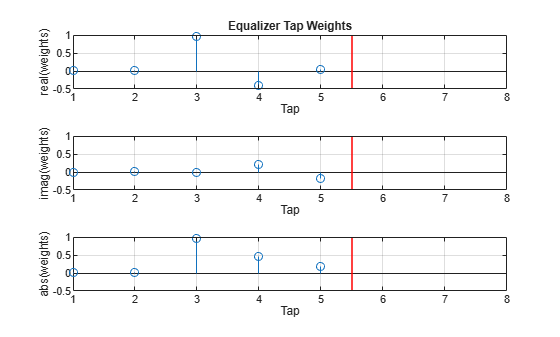expand all

expand all